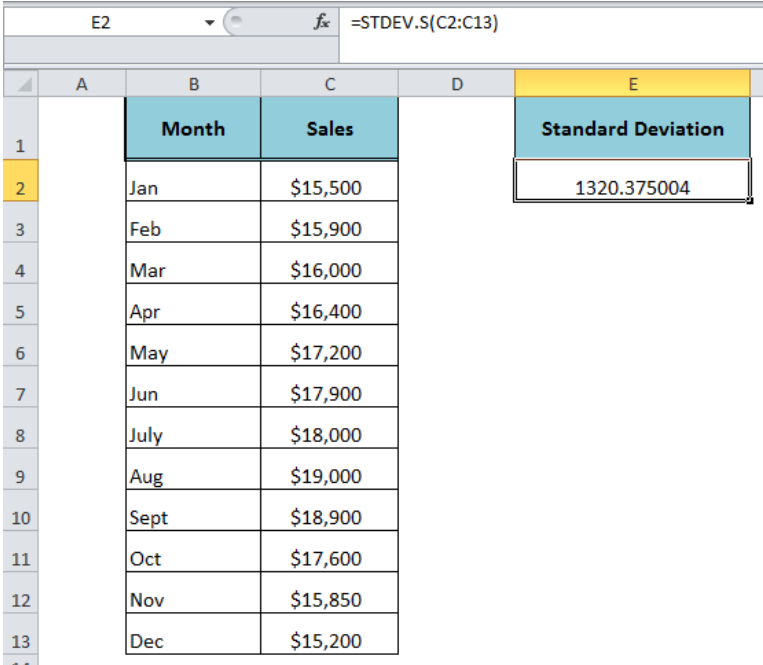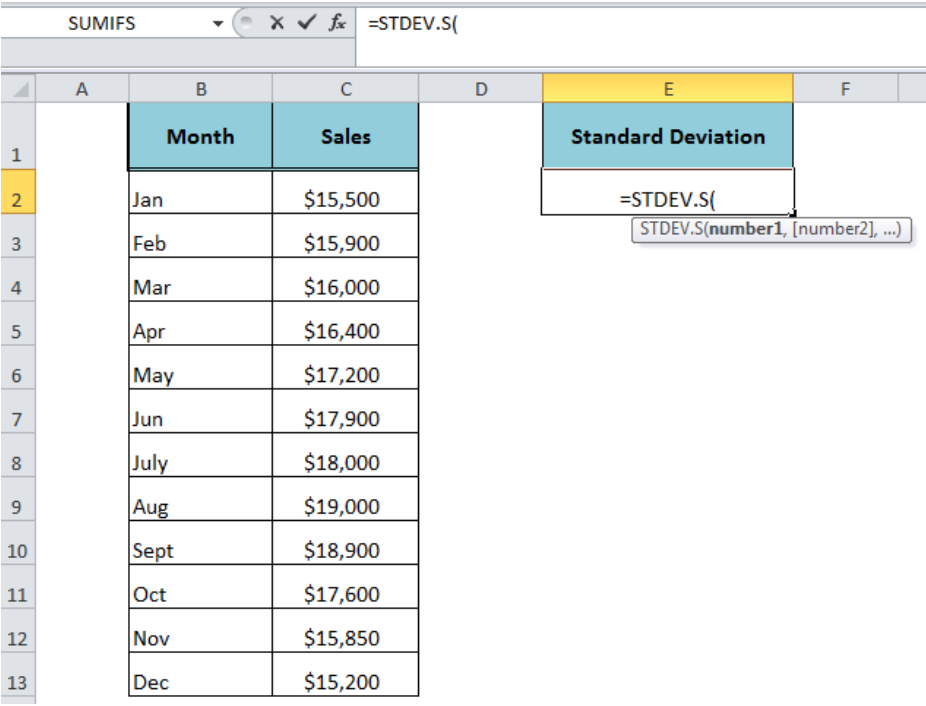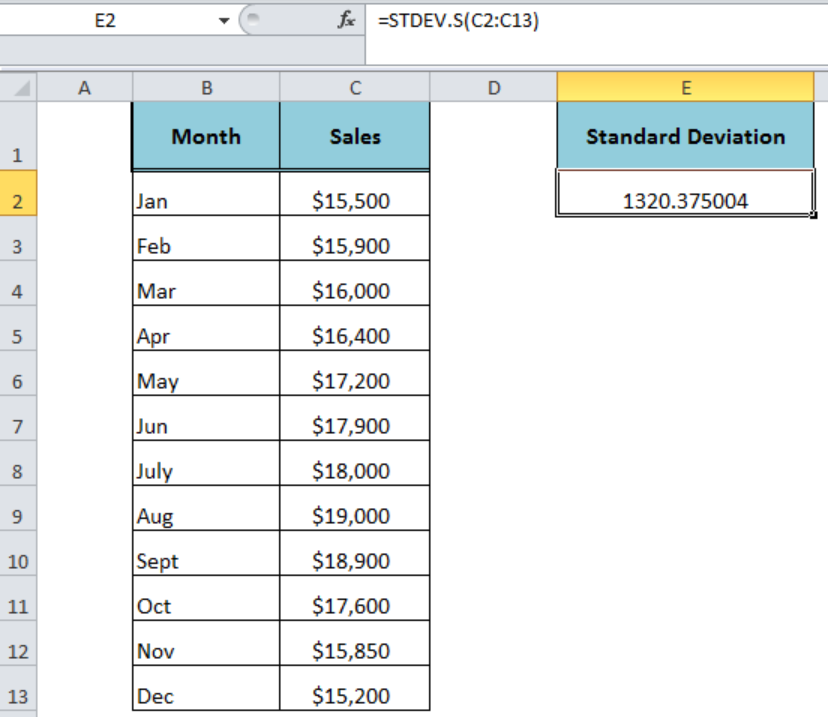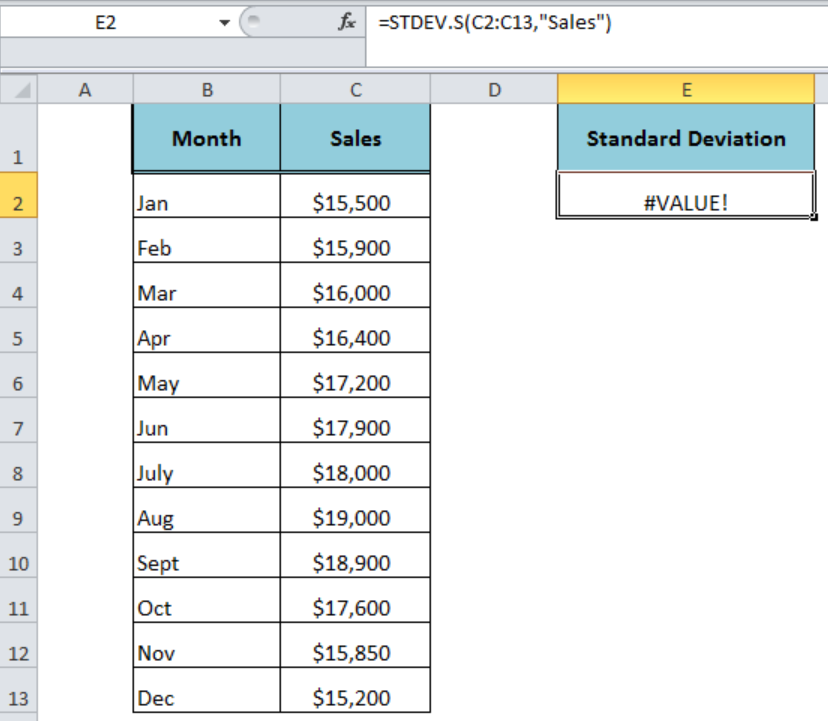Get instant live expert help with Excel or Google Sheets“My Excelchat expert helped me in less than 20 minutes, saving me what would have been 5 hours of work!”

#### Post your problem and you’ll get Expert help in seconds.

Your message must be at least 40 characters
Our professional Expert are available now. Your privacy is guaranteed.

# Excel STDEV.S Function

The Excel STDEV.S function calculates the standard deviation of a set of numbers that represents a sample. It determines how much the values of sample dataset are widely spread compared to the average of the sample numbers.Figure 1. How to Use the Excel STDEV.S Function

## Syntax

The syntax for the STDEV.S function is as follows;

`=STDEV.S(number1,[number2],...)`

It can accept the arguments between 1 and 255 in total.

• number1 – It is the required argument and the first number of the sample.
• number2,… –  (Optional) These are additional arguments of sample numbers between 2 and 255.

The STDEV.S function supports arguments as constant numbers, the text representation of numbers, cell references, ranges or arrays. It ignores the text, logical values (TRUE and FALSE), empty cells and text representations of numbers in the range, array or cell references and evaluates only numbers of sample dataset in the calculation. However, if the logical value and text representations of numbers are supplied directly as the argument in the STDEV.S function then it evaluated these values as numbers.Figure 2. The Syntax For the STDEV.S Function

## Example

Let say we have a sample of monthly sales in range C2: C13. We want to estimate the standard deviation of the sample monthly sales to figure out how much monthly sales values have variance from the average of these sample sales values. We need to use the following formula in cell E2 to calculate the standard deviation of sample monthly sales;

`=STDEV.S(C2: C13)`Figure 3. The Formula Output of the STDEV.S Function

## Usage Notes

• The Excel STDEV.S function has been introduced in Excel 2010 to replace the STDEV function. The STDEV function is still available for compatibility with Excel 2007 and earlier versions.
• If all of the supplied arguments contain only text values then it generates #DIV/0! error.
• If a text value is entered directly in the argument then it generates #VALUE! error.Figure 4. The #VALUE! Error Value of the STDEV.S Function

## Instant Connection to an Expert through our Excelchat Service:

Most of the time, the problem you will need to solve will be more complex than a simple application of a formula or function. If you want to save hours of research and frustration, try our live Excelchat service! Our Excel Experts are available 24/7 to answer any Excel question you may have. We guarantee a connection within 30 seconds and a customized solution within 20 minutes.

Solution examplesI need to count number of dates from a timestamped date in column from the completed date column to show the number of days. I have not successfully been able to do this. I am a novice Excel user. Can you help?
Solved by O. B. in 15 minsUse the Vlookup Function to complete the "employee" column of table 2. Use "job Id" from table 2 as your lookup_value(s) and table 1 as your reference.
Solved by C. H. in 16 minsIf a cell in another sheet is populated I need a vlookup done. If the cell is not populated I need the cell to return blank.
Solved by T. D. in 60 minsI am trying to make a chart that turns a week range red if nothing is entered in the range. If something is entered then I would like it to turn green. Please Help
Solved by E. U. in 43 minsI need a check box to show/hide an answer of an if function
Solved by Z. U. in 23 mins